#### Chapter 6 Factorization of Polynomials R.D. Sharma Solutions for Class 9th Math Exercise 6.1

Exercise 6.1

1. Which of the following expressions are polynomials in one variable and which are not? State reasons for your answer:Solution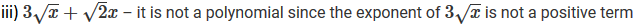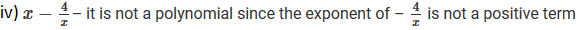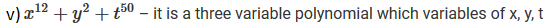Solution3. Write the degrees of each of the following polynomials:Solution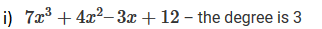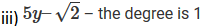4. Classify the following polynomials as linear, quadratic, cubic and biquadratic polynomials:Solution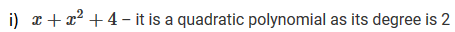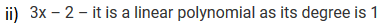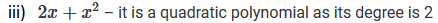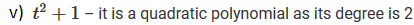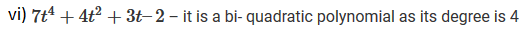5. Classify the following polynomials as polynomials in one-variable, two variables etc.:Solution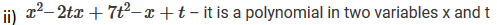6. Identify polynomials in the following:Solution

(i) It is a polynomial. The power of variable of all 'x' is positive integer.
(ii) It is not a polynomial since the exponent of √x is a negative integer.
(iii) It is a polynomial. The power of variable of all 'x' is positive integer.
(iv) It is not a polynomial since the exponent of 4/x is a negative integer.
(v) It is a polynomial. The power of variable of all 'x' is positive integer.
(vi) It is not a polynomial. Since the exponent of 3/x is a negative integer.

7. Identify constant, linear, quadratic and cubic polynomials from the following polynomials:Solution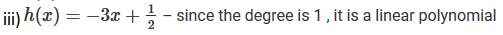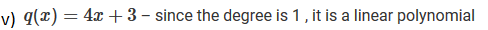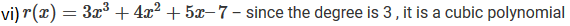8. Give one example each of a binomial of degree 35, and of a monomial of degree 100.
Solution
Example of a binomial with degree 35: 6x35-25
Example of a monomial with degree 100: 3z100